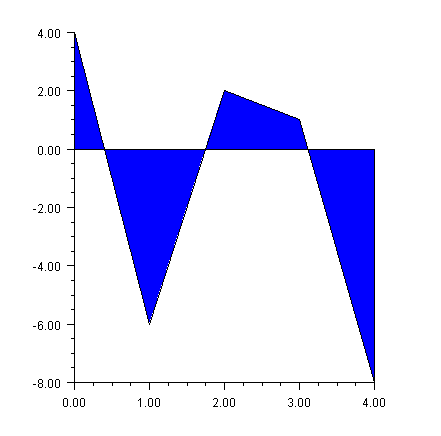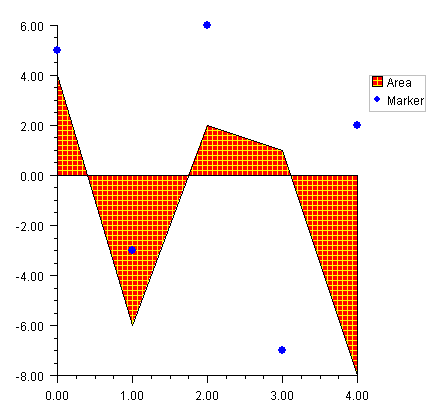JMSL Chart Programmer’s Guide
Area Plot
Area plots are similar to line plots, but with the area between the line and a reference line filled in. An area plot is created if the DATA_TYPE_FILL bit is on in the value of the DataType attribute. The default reference line is y =0. The location of the reference line can be changed from 0 by using setReference(double). The Fill Area Attributes determine how the area is filled.
Simple Area Plot
This example draws a simple area plot. The default value of the FillType attribute is FILL_TYPE_SOLID. The example sets the FillColor attribute to blue. So the area between the line and y = 0 is solid blue.View code file

import com.imsl.chart.*;
import java.awt.Color;

public class SampleArea extends JFrameChart {

public SampleArea() {
Chart chart = getChart();
AxisXY axis = new AxisXY(chart);
double y[] = {4, -6, 2, 1, -8};
Data data = new Data(axis, y);
data.setDataType(Data.DATA_TYPE_FILL);
data.setFillColor(Color.blue);
}
public static void main(String argv[]) {
new SampleArea().setVisible(true);
}
}
Painted Area Example
This example shows an area chart filled in with a painted texture. The texture is created by a static method FillPaint.crosshatch.
A second data set is plotted as a set of markers.
The Legend node is painted in this example and has entries for both the filled area data set and the marker data set.View code file

import com.imsl.chart.*;
import java.awt.Color;

public class SampleAreaPaint extends JFrameChart {

public SampleAreaPaint() {
Chart chart = getChart();
AxisXY axis = new AxisXY(chart);
chart.getLegend().setPaint(true);
double y1[] = {4, -6, 2, 1, -8};
Data data1 = new Data(axis, y1);
data1.setTitle("Area");
data1.setDataType(Data.DATA_TYPE_FILL);
data1.setFillType(Data.FILL_TYPE_PAINT);
data1.setFillPaint(FillPaint.crosshatch
(10,5,Color.red,Color.yellow));
double y2[] = {5, -3, 6, -7, 2};
Data data2 = new Data(axis, y2);
data2.setTitle("Marker");
data2.setDataType(Data.DATA_TYPE_MARKER);
data2.setMarkerColor(Color.blue);
data2.setMarkerType(Data.MARKER_TYPE_FILLED_CIRCLE);
}
public static void main(String argv[]) {
new SampleAreaPaint().setVisible(true);
}
}
Attribute Reference
The attribute Reference defines the reference line. If its value is a, then the reference line is y = a. Its default value is 0.